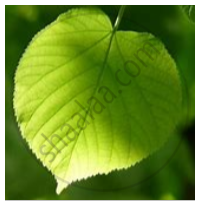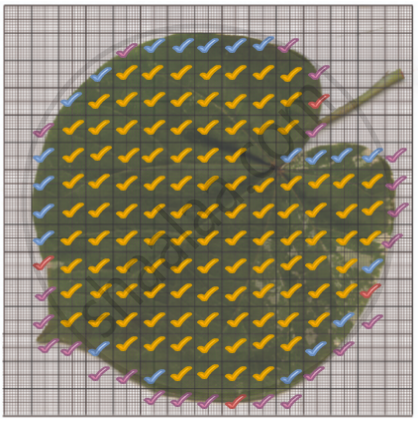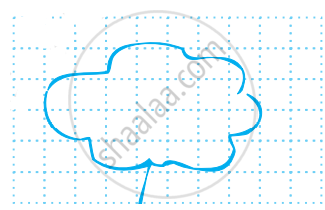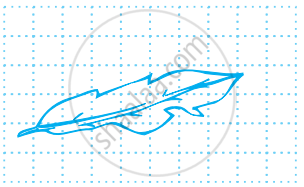# Concept of Area

#### definition

Area: The amount of surface enclosed by a closed figure is called its area.

# Area:

• The amount of surface enclosed by a closed figure is called its area.

• A polygon is a plane shape with straight sides. The area of a polygon measures the size of the region enclosed by the polygon.

• It is measured in units squared.

## Area of Regular Shapes

Area of shapes such as circle, triangle, square, rectangle, parallelogram, etc. are the region occupied by them in space. Polygon shape: A polygon is a two-dimensional shape that is formed by straight lines. The examples of polygons are triangles, hexagons and pentagons.

Area of Square

Area of Rectangle

Area of Triangle

## Visualisation of covered Area on the graph:It is difficult to tell the area of the figure just by looking at the figure.

Place them on a squared paper or graph paper where every square measure 1 cm × 1 cm.

Make an outline of the figure.

Look at the squares enclosed by the figure. Some of them are completely enclosed, some half, some less than half and some more than half.

The area is the number of centimeter squares that are needed to cover it

But there is a small problem: the squares do not always fit exactly into the area you measure. We get over this difficulty by adopting a convention:

• The area of one full square is taken as 1 sq unit. If it is a centimeter

• square sheet, then the area of one full square will be 1 sq cm.

• Ignore portions of the area that are less than half a square.

• If more than half of a square is in a region, just count it as one square.

• If exactly half the square is counted, take its area as 1/2sq unit.

Such a convention gives a fair estimate of the desired area.Covered area Number Area estimate (sq. units) (i) Fully-filled squares 101 101 (ii) Half-filled squares 4 4 × 1/2 (iii) More than half-filled squares 21 21 (iv) Less than half-filled squares 23 0

Total area = 101 + 4 × 1/2 + 21 = 1261/2 sq. Units.

#### Example

By counting squares, estimate the area of the figure.Covered area Number Area estimate (sq units) (i) Fully-filled squares 11 11 (ii) Half-filled squares 3 3 xx 1/2 (iii) More than half-filled squares 7 7 (iv) Less than half-filled squares 5 0

Total area = 11 + 3 × 1/2 + 7 = 19 1/2 sq units.

#### Example

By counting squares, estimate the area of the figure.Covered area Number Area estimate (sq units) (i) Fully-filled squares 1 1 (ii) Half-filled squares - - (iii) More than half-filled squares 7 7 (iv) Less than half-filled squares 9 0

Total area = 1 + 7 = 8 sq units.

If you would like to contribute notes or other learning material, please submit them using the button below.

### Shaalaa.com

Area Part-1 [00:21:56]
S
0%